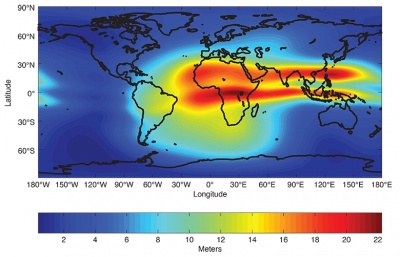If you wish to contribute or participate in the discussions about articles you are invited to contact the Editor

# NeQuick Ionospheric ModelFundamentals
Title NeQuick Ionospheric Model
Author(s) J. Sanz Subirana, J.M. Juan Zornoza and M. Hernández-Pajares, Technical University of Catalonia, Spain. [Updated 2017]: R. Prieto-Cerdeira, R. Orús Pérez, European Space Agency, ESA/ESTEC.
Level Intermediate
Year of Publication 2011, updated 2017

NeQuick-G is the ionospheric model adopted by the Galileo system in order to help to compute the ionospheric delay corrections for its single-frequency users [Galileo Open Service-Ionospheric Correction Algorithm, 2016] . The NeQuick-G model is an adaptation for real-time users of the ITU-R NeQuick ionospheric electron density model [ITU-R, 2013]  based on the original profiler proposed by [Di Giovanni and Radicella, 1990] [Hochegger, Nava et al., 2000].NeQuick a three-dimensional and time dependent ionospheric electron density model based on an empirical climatological representation of the ionosphere, which predicts monthly mean electron density from analytical profiles, depending on solar-activity-related input values: sun spot number or solar flux, month, geographic latitude and longitude, height and Universal Time (UT).

NeQuick model has been adapted for real-time Galileo single-frequency ionospheric corrections (for convenience referred to as NeQuick-G) in order to derive real-time predictions based on a single input parameter, the Effective ionisation level, Az, which is determined using three coefficients broadcast in the navigation message. As a three-dimensional and time-dependent model, it has the capability to provide vertical or slant ionospheric delay correction by integrating the predicted electron density along the line of sight vector between satellite and receiver.

In order to take into account both daily variation of the solar activity and the user’s local geomagnetic condition, the NeQuick-G model is driven by and computes its correction by use of the daily solar activity information, so-called effective ionization level (Az) expressed in solar flux unit (10-22Wm-2Hz-1)

For Galileo single-frequency users, Galileo satellites broadcast three ionospheric coefficients in their navigation message that are used to compute the Az as follows [Galileo - Open Service SIS-ICD, 2016] :

$\displaystyle{ Az=a_0+a_1\mu + a_2 \mu^2 \qquad\mbox{(1)} }$

where $\displaystyle{ \mu }$ is the modified dip latitude, or MODIP:

$\displaystyle{ \tan \mu=\displaystyle \frac{I}{\sqrt{\cos \phi}}\qquad\mbox{(2)} }$

being $\displaystyle{ I }$ the true magnetic inclination[footnotes 1], or dip in the ionosphere (usually at 300 km), and $\displaystyle{ \Phi }$ the geographic latitude of the receiver [Rawer, 1963].

The coefficients $\displaystyle{ a_0 }$, $\displaystyle{ a_1 }$,$\displaystyle{ a_2 }$ will be broadcasted to the users in the Galileo navigation message and updated at least once a day [footnotes 2].Figure 1: Global vertical Total Electron Content map generated with NeQuick G model at 13h UT for a day in April and Az=193.

The Galileo single frequency receiver algorithm will be based in the following steps (the next paragraph is taken from [Arbesser-Rastburg, B., 2006]):

1. $\displaystyle{ Az }$ is evaluated using $\displaystyle{ a_0 }$, $\displaystyle{ a_1 }$,$\displaystyle{ a_2 }$ (from navigation message) and MODIP from the NeQuick model (which depends on estimated receiver position).
2. Electron density is calculated for a point along the satellite to receiver path, using the NeQuick model with $\displaystyle{ Az }$ in place of $\displaystyle{ F10.7 }$.
3. Steps 1 and 2 are repeated for many discrete points along the satellite receiver path. The number and spacing of the points will depend on the height and they will be a trade-off between integration error and computational time and power.
4. All electron density values along the ray are integrated in order to obtain Slant TEC (STEC).
5. Slant TEC, in TECUs, is converted to meters of L1 slant delay for correcting pseudo-ranges, by
$\displaystyle{ I_f = \frac{40.3\cdot 10^{16}}{f^2}TEC \qquad\mbox{(where f is in Hz)} \quad\mbox{(3)} }$

An overview of the correction algorithm and initial performance results with broadcast parameters from Galileo satellites have been performed during IOV in the period April 2013 to March 2014 during solar maximum of Solar Cycle 24 [Prieto-Cerdeira, Orús-Pérez et al, 2014] .

Notice that, as with the Klobuchar model, the ionospheric corrections computed by the NeQuick G can be used for any GNSS signal (GPS, GLONASS, Galileo…) simply by setting the corresponding frequency in the equation (3).